# How to Calculate VAT Tax – Excel & Google Sheets

This tutorial will demonstrate how to calculate VAT in Excel and Google Sheets.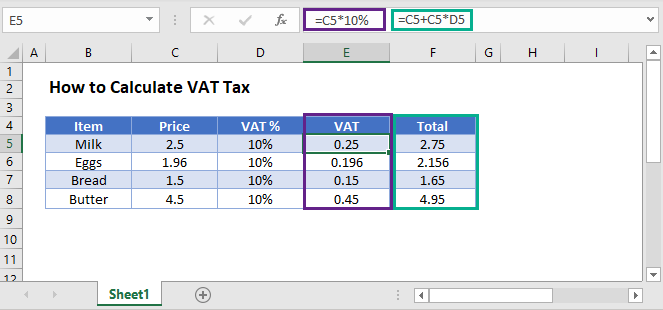## Calculate VAT in Excel

VAT can be calculated in Excel using a simple formula.

``=C5*10%``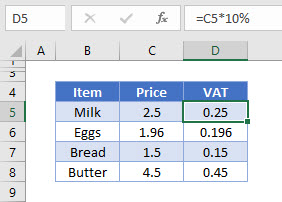This formula will calculate the VAT amount based on a VAT rate of 10%. When you enter the formula, you can either type 10% or you can type 0.1 – both methods will return the same result.

To get the total value of the product, you would then need to add the Price + VAT together

``=C5+D5``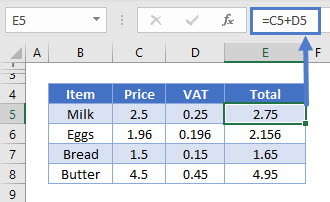You can also calculate the VAT in a formula without having a separate VAT column in your spreadsheet.

This formula will return a total price including the VAT amount.

``=C5+(C5*10%)``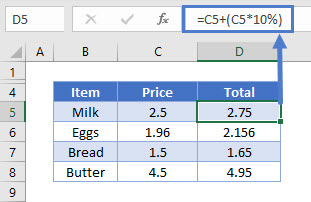## Calculate VAT in Google Sheets

You can calculate VAT in Google Sheets in the same way.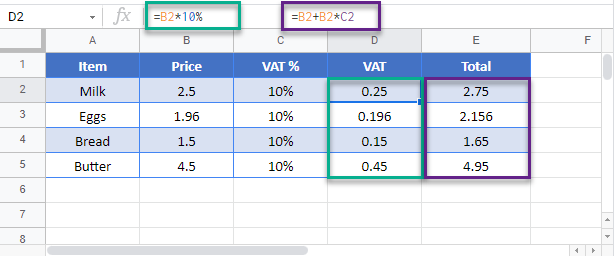### Excel Practice Worksheet

Practice Excel functions and formulas with our 100% free practice worksheets!